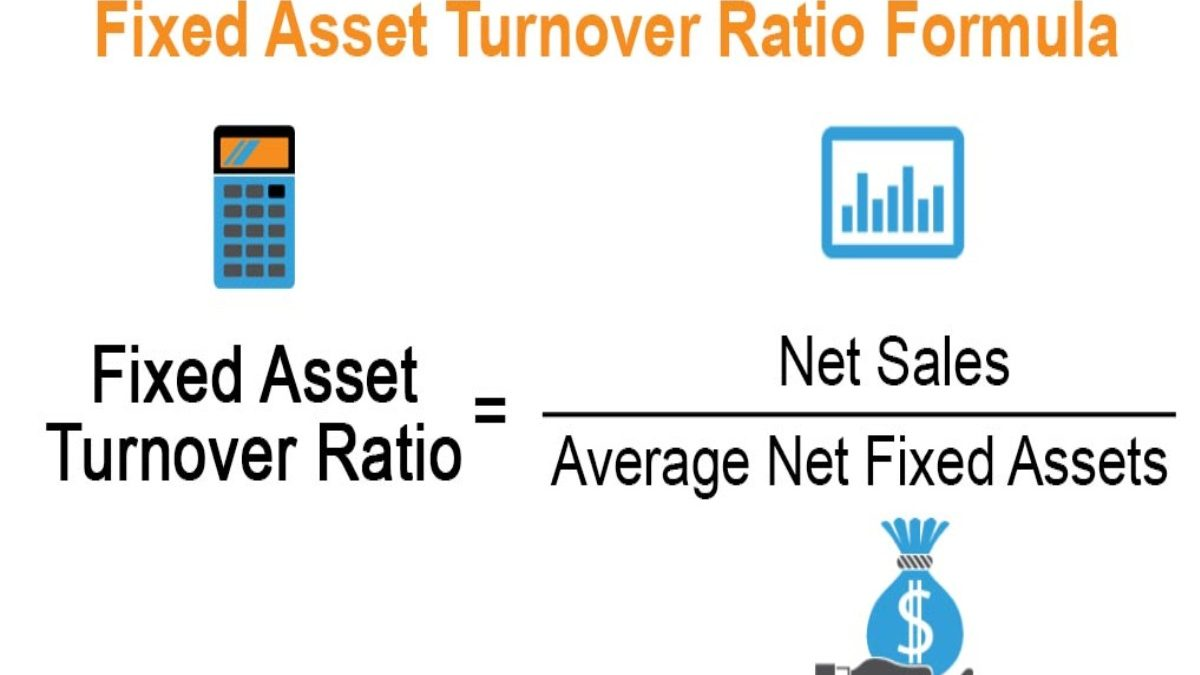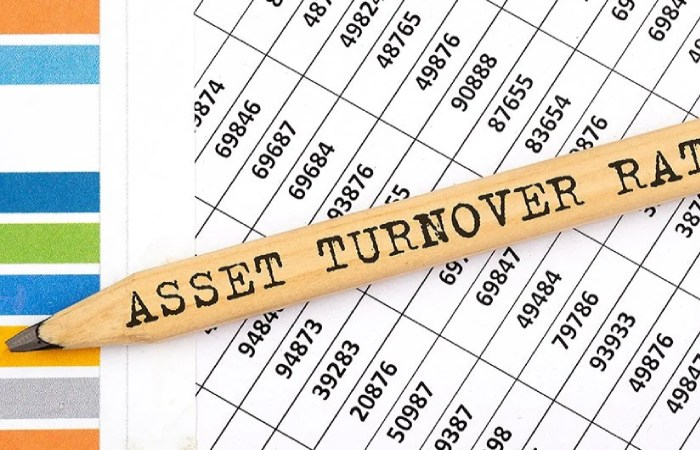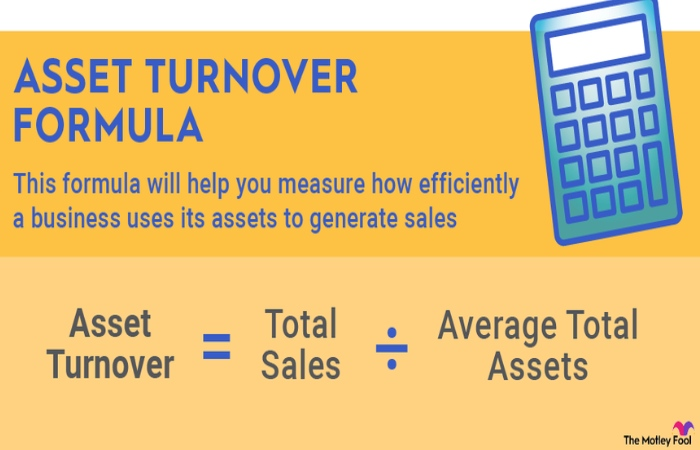29 Sep 2023

## Blog Post# Asset Turnover Ratio Formula – 2023

## Introduction

Asset Turnover Ratio Formula: Any company’s success is mainly created by using its assets to generate sales effectively. The asset turnover ratio measures the efficiency with which a company uses its assets to make sales by comparing the value of its sales revenue relative to the average value of its assets.

Companies depend on their working capital to generate sales revenue. Working capital consists of a company’s cash flow and assets. The asset turnover ratio measures how efficiently a company utilizes its help to produce sales revenues.

## Asset Turnover Ratio Formula & CalculationThe formula for calculating the asset turnover ratio consists of two factors:

1. A company’s net sales: its gross sales after accounting for returns, discounts, and allowances
2. Its total assets: the total of its equity and liabilities at the beginning of the year plus the total at the end of the year divided by two.

The formula expressed as:

Asset Turnover Ratio = Net Sales/Total Assets

## Asset Turnover Ratio Formula

Let us look at our 1st example.

1. Take Company A and Company B for calculating the ratio.
2. Values given in the example below are in \$ millions.
3. Consider below-given income statement for both companies.
4. To calculate the ratio, we need
5. Net Sales
6. Average total assets

Let us calculate Net sales for both companies

Company A

• Sales = \$ 2,000
• Sales return = \$ 200
• Net sales = \$1,800

Company B

• Revenue = \$3,000
• Sales return =\$150
• Net sales = \$2,850

Now, consider the below-given Balance Sheets for both companies.

Let us calculate the Average total assets for both companies.

In the given example, we have total assets for only one period. Hence the same can be used as the Average total assets.

### Average total assets

Company A = \$6,253

Company B =\$3,923

Now that we know all the values, let us calculate the ratio for both companies.

Asset turnover ratio = Net Sales / Average total asset

• Company A = \$1,800/ \$6,253 = 0.28 x
• Company B = \$2,850/ \$3,923 = 0.72 x

Hence, the ratio for both companies is below one time.

What this mean is that companies are not managing their overall asset efficiently.

They cannot generate revenue that is at least equal to their asset base.

Nonetheless, Company B is relatively more efficient in utilizing its assets to generate revenue when compared to Company A.

## Calculating the Total Asset Turnover1. Gather information. You will need to gather some information to calculate your total asset turnover. Suppose you do not know your net sales and average whole asset numbers. In that case, you must have the information to determine your net sales and average total assets. Use your business spreadsheets to help you resolve these numbers.
2. Determine the amount of net sales. It would be best to subtract the year’s total allowances and discounts to determine your net sales. For example, if you own a clothing store and sold \$200,000 of clothing the previous year but had \$10,000 in returns and gave \$5,000 in discounts. Your net sales for the year would be \$185,000.

total sales – (returns + allowances) = net sales

1. Determine the amount of average total assets. You must go back two years in your bookkeeping to determine your average total assets. Find the number of your full support for the current and previous years, then add the two numbers together. Next, divide the total amount by 2. For example, if your real asset at the end of the previous year was \$275,000 and your entire investment at the current year was \$237,000, the total amount would be \$512,000. When you divide \$512,000 by 2, your total is \$256,000. Therefore, \$256,000 would be your average total asset number.

(total assets for previous year + total assets for current year) / 2

1. Calculate the total asset turnover. Now that you have your net sales and average total asset numbers, you can calculate your total asset turnover. To calculate your total asset turnover, you must divide your net sales by your average total asset number. Use the total asset turnover ratio:

total asset turnover = net sales / average total assets

Our hypothetical calculation would be: \$185,000 / \$256,000 = 0.72

The asset turnover ratio has wide importance for investors and may influence their investing decisions. Read further to know about the advantages.

• Measure company performance – The asset turnover ratio used to analyze a company’s performance. And as investors constantly look for investment opportunities, a high asset turnover ratio could attract new investors.
• Saves extra expenditure– Management should consider an asset’s advantages before purchasing it. It can help cut down on wasteful spending.
• Facilitate comparison– Investors use a measure to compare one company with another within the same sector before any decision-making.
• Indication of asset impairment– The ratio can indicate asset impairment. For instance, a sudden drop in the ratio may indicate that the company assets are losing their ability to generate income.

## Limitations of Assets Turnover Ratio

1. The asset turnover ratio does not offer all the information necessary for thorough research.
2. It does not exclude the idle assets not employed in the production process, providing an inaccurate result.
3. The asset turnover ratio of a company may vary significantly for different accounting periods, making comparison difficult.
4. When a business makes important asset purchases expecting faster growth, the asset turnover ratio might be unnaturally lower.
5. Similar selling off assets may unnaturally raise the percentage. The asset turnover ratio of a company thus remains obstructed by various factors and cannot be taken at face value.

## Asset Turnover Ratio Example

Consider the net sale and total assets of two illusory retail companies as an example of how the asset turnover ratio is applied.

 Company X Company Y Net Sales (Revenue) \$460,000,000 \$70,000,000 Beginning Assets \$200,000,000 \$40,000,000 Ending Assets \$195,000,000 \$39,000,000 Average Total Assets \$197,500,000 \$39,500,000 Asset Turnover Ratio 2.33 1.77

The asset turnover ratio for these two retail companies provides a straight-across evaluation of their performance.

Company X generated \$2.33 in sales per dollar of assets.

Company Y generated \$1.77

It shows that company X is more efficient in using assets to produce revenue. The lower ratio for Company Y may indicate sluggish sales or carrying too much obsolete inventory. It could also result from investments, such as property or equipment, not utilized to their optimum capacity.

## Asset Turnover Ratio vs. Fixed Asset Turnover

Asset management ratios such as asset turnover ratio are critical to analyzing how the company manages its asset to generate revenue. Some investors or analysts are more concerned with how the company works its fixed assets and the efficiency they use to generate sales revenue. Whereas the asset turnover ratio looks at a company’s total assets, the fixed asset ratio only considers its fixed assets. Fixed assets are reported on the balance sheet net of accumulated depreciation and can include the following:

• Property
• Plants
• Equipment

While the fixed asset ratio is also an efficiency measure of a company’s operating performance, it is widely used in manufacturing companies that rely heavily on plant and equipment. As with the asset turnover ratio, the fixed asset turnover ratio measures operational efficiency. However, it is less likely to fluctuate because fixed asset values are more static. Companies with high fixed asset ratios tend to be well-managed and more effective at utilizing their investment in fixed assets to produce sales.

Key Takeaway: While the asset turnover and fixed asset turnover ratios are similar, the fixed asset ratio is more meaningful for companies with significant investments in property, plants, and equipment.

## Conclusion

It can use to gauge the company’s efficiency and profitability. This formula use two variables: Annual revenue and average cash balance. The high Cash Turnover Ratio indicates that company is taking the shortest time possible to refresh its cash account using its sale revenue.